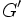# Perfect group

VIEW RELATED: Analogues of this | Variations of this | Opposites of this |
View a complete list of semi-basic definitions on this wiki
This article defines a group property that is pivotal (i.e., important) among existing group properties
View a list of pivotal group properties | View a complete list of group properties [SHOW MORE]

## Definition

A group is said to be perfect if it satisfies the following equivalent conditions:

No. Shorthand A group is said to be perfect if ... A group$G$ is said to be perfect if ...
1 equals own derived subgroup it equals its own derived subgroup (i.e., its commutator subgroup with itself).$G$ equals the derived subgroup$[G,G]$, sometimes also denoted$G'$.
2 every element is a product of commutators every element of the group can be expressed as a product in the group of finitely many elements each of which is a commutator. for any$g \in G$, there exist elements$g_1,g_2,\dots,g_{2n-1},g_{2n}$ (with possible repetitions) such that$g = [g_1,g_2][g_3,g_4] \dots [g_{2n-1},g_{2n}]$.
3 trivial abelianization its abelianization is a trivial group. the abelianization$G^{\operatorname{ab}} = G/[G,G] = H_1(G;\mathbb{Z})$ is the trivial group.
4 trivial homomorphism to any abelian group any homomorphism of groups from it to an abelian group is the trivial homomorphism. for any abelian group$K$, and any homomorphism of groups$\varphi:G \to K$,$\varphi$ must send all elements of$G$ to the identity element of$K$.
This definition is presented using a tabular format. |View all pages with definitions in tabular format

### In terms of the fixed-point operator

The property of being perfect is obtained by applying the fixed-point operator to a subgroup-defining function, namely the derived subgroup.

## Examples

### Groups satisfying the property

Here are some basic/important groups satisfying the property:

Here are some relatively less basic/important groups satisfying the property:

GAP ID
Alternating group:A560 (5)
Alternating group:A6360 (118)
Projective special linear group:PSL(3,2)168 (42)
Special linear group:SL(2,5)120 (5)

Here are some even more complicated/less basic groups satisfying the property:

GAP ID
Alternating group:A7
Projective special linear group:PSL(2,11)660 (13)
Projective special linear group:PSL(2,8)504 (156)
Special linear group:SL(2,7)336 (114)
Special linear group:SL(2,9)720 (409)

### Groups dissatisfying the property

Note that any nontrivial solvable group cannot be a perfect group, so this gives lots of non-examples. The examples discussed below concentrate more on the non-solvable groups that still fail to be perfect.

Here are some basic/important groups that do not satisfy the property:

Here are some relatively less basic/important groups that do not satisfy the property:

GAP ID
Symmetric group:S5120 (34)

Here are some even more complicated/less basic groups that do not satisfy the property:

## Metaproperties

Metaproperty name Satisfied? Proof Statement with symbols
subgroup-closed group property No perfectness is not subgroup-closed It is possible to have a perfect group$G$ and a subgroup$H$ of$G$ such that$H$ is not perfect. In fact, any nontrivial perfect group has a nontrivial cyclic subgroup that is not perfect. (in fact, every finite group is a subgroup of a finite perfect group).
characteristic subgroup-closed group property No perfectness is not characteristic subgroup-closed It is possible to have a perfect group$G$ and a characteristic subgroup$H$ of$G$ such that$H$ is not perfect.
quotient-closed group property Yes perfectness is quotient-closed If$G$ is a perfect group and$H$ is a normal subgroup of$G$, the quotient group$G/H$ is also a perfect group.
finite direct product-closed group property Yes perfectness is finite direct product-closed Suppose$G_1,G_2,\dots,G_n$ are all (possibly isomorphic, possibly non-isomorphic) perfect groups. Then, the external direct product$G_1 \times G_2 \times \dots \times G_n$ is also a perfect group.
join-closed group property Yes perfectness is join-closed Suppose$H_i, i \in I$ is a (possibly finite, possibly infinite) collection of subgroups of a group$G$, such that each$H_i$ is a perfect group. Then, the join of subgroups$\langle H_i \rangle_{i \in I}$ is a perfect group.

## Relation with other properties

### Stronger properties

Property Meaning Proof of implication Proof of strictness (reverse implication failure) Intermediate notions
superperfect group perfect and Schur-trivial: the Schur multiplier is trivial. (by definition) follows from perfect not implies Schur-trivial |FULL LIST, MORE INFO
simple non-abelian group non-abelian and has no proper nontrivial normal subgroup. simple and non-abelian implies perfect Quasisimple group, Semisimple group|FULL LIST, MORE INFO
quasisimple group perfect and inner automorphism group is a simple non-abelian group Semisimple group|FULL LIST, MORE INFO
group in which every element is a commutator every element of the group is a commutator of two elements of the group. |FULL LIST, MORE INFO

### Weaker properties

Property Meaning Proof of implication Proof of strictness (reverse implication failure) Intermediate notions
square-in-derived group every square element is in the derived subgroup. |FULL LIST, MORE INFO
stem group the center is contained in the derived subgroup |FULL LIST, MORE INFO
group with unique Schur covering group the Schur covering group is unique, or equivalently,$\operatorname{Ext}^1$ of the abelianization over the Schur multiplier is trivial. |FULL LIST, MORE INFO

## Testing

### GAP command

This group property can be tested using built-in functionality of Groups, Algorithms, Programming (GAP).
The GAP command for this group property is:IsPerfectGroup
View GAP-testable group properties

To test whether a given group is perfect, the command is:

IsPerfectGroup(group);

The command:

PerfectGroup(n,r)

gives the$r^{th}$ perfect group of order$n$. If$r$ is not specified, this simply gives the first perfect group of order$n$. An error is thrown if there are no perfect groups of order$n$.